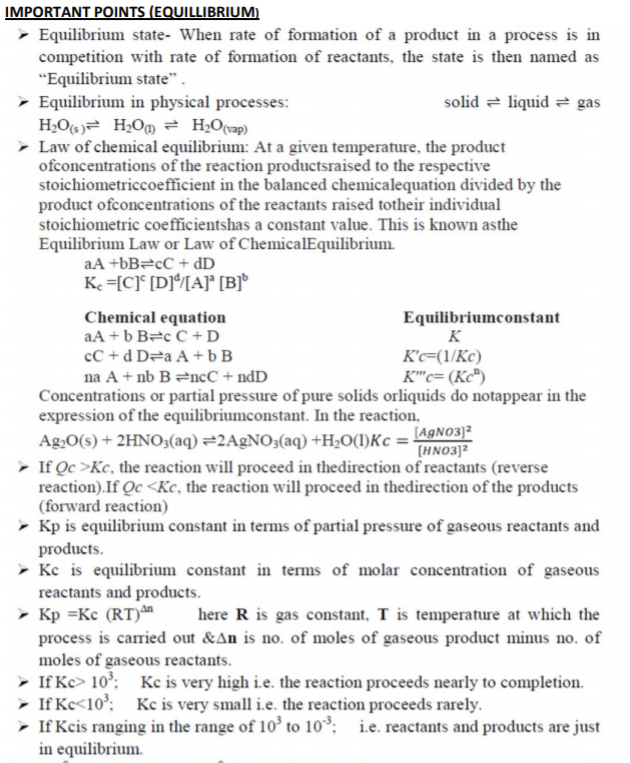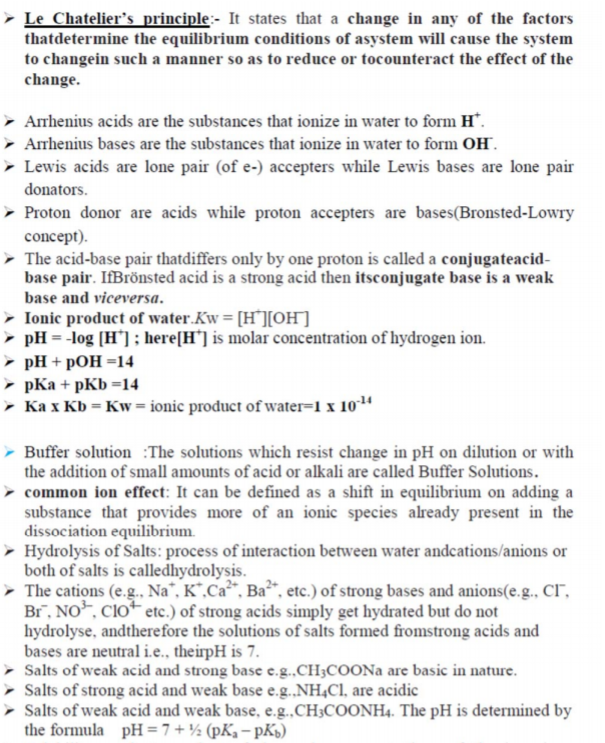# CBSE Class 11 Chemistry Equilibrium Notes Set E

Download CBSE Class 11 Chemistry Equilibrium Notes Set E in PDF format. All Revision notes for Class 11 Chemistry have been designed as per the latest syllabus and updated chapters given in your textbook for Chemistry in Standard 11. Our teachers have designed these concept notes for the benefit of Grade 11 students. You should use these chapter wise notes for revision on daily basis. These study notes can also be used for learning each chapter and its important and difficult topics or revision just before your exams to help you get better scores in upcoming examinations, You can also use Printable notes for Class 11 Chemistry for faster revision of difficult topics and get higher rank. After reading these notes also refer to MCQ questions for Class 11 Chemistry given our website

## Revision Notes for Class 11 Chemistry Equilibrium

Class 11 Chemistry students should refer to the following concepts and notes for Equilibrium in standard 11. These exam notes for Grade 11 Chemistry will be very useful for upcoming class tests and examinations and help you to score good marks

### Equilibrium Notes Class 11 ChemistryIMPORTANT QUESTIONS CHAPTER‐ EQUILIBRIUM

1. Equilibrium constant helps in predicting the direction in which a given reaction will proceed at any stage.

a) In which one of the following conditions a chemical reaction Proceeds in the forward direction?

i) QC < KC

ii) QC > KC

iii) QC = 1/KC

IV) QC = ‐ KC

b) Write whether the following statement is true or false:

"High value of equilibrium constant suggests high concentration of the reactants in the equilibrium mixture”.

c) State the Le‐Chatliers principle. Applying this principle, explain the effect of pressure in the following

CO(g) + 3 H2(g) → CH4(g) + H2O(g) (3)

2. a) i) Give the Arrhenius concept about acids and bases.

ii) Give one example each for Arrhenius acid and

b) i) Write the expression for equilibrium constant Kp for the following

2NOCl(g) 2NO(g) + Cl2(g)

ii) Find the value of Kc for the above equilibrium if the value of Kp is 8 x 10‐2 atm at 600 K. (R= 0.0821 Latm K‐1mol‐1)

3. Le‐Chatlier’s principle makes a qualitative prediction about the change in conditions on equilibrium.

a) State Le‐Chatlier’s principle.

b) N2(g) + O2(g) 2NO(g).

What is the effect of pressure on the above equilibrium?

c) The species HCO3‐ and HSO4‐ can act both as Bronsted acids and bas Write the corresponding conjugate acid and conjugate base of the above species.

4.a) Write an equation for equilibrium constant in terms of concentration (Kc) for the equilibrium reaction given below.

Ag2O(s) + 2HNO3(aq) 2 AgNO3(aq) + H2O(l) (1)

b) What are buffer solutions? Give an example for a buffer solution

c) The concentration of H+ ion in a sample of soft drink is 3.8 x 10‐3M. Determine its pH.

4.a) What is conjugate acid – base pair? Illustrate with an example.

b) Define the pH The pH of a soft drink is 2.42. Give the nature of the solution.

c) An aqueous solution of CuSO4 is acidic while that of Na2SO4 is neutral. Explain.

5. Equilibrium is possible only in a closed system at a given temperature.

6. a) Write the expression for equilibrium constant, Kc for the reaction

4 NH3(g) + 5 O2(g) 4 NO(g) + 6 H2O(l)

b) What happens to the value of the equilibrium constant (Kc) when the above reaction is reversed?

7. Weak acids are partially ionized in aqueous solutions.

a) The ionization constants of some acids are given below: Acid Ionisation constant (Ka)

Formic acid (HCOOH) 1.8 x 10‐4

Hypochlorous acid (HClO) 3.0 x 10‐8

Nitrous acid (HNO2) 4.5 x 10‐4

Hydrocyanic acid (HCN) 4.9 x 10‐10

Arrange the above acids in the increasing order of their acid strength.

b) Calculate the pH of a 01 M acetic acid solution with the degree of ionization 0.045.

8. Salts can be classified into different categories on the basis of their solubility.

a) Identify the solubility range of sparingly soluble salts from the following:

(Between 0.01 M and 0.1 M, less than 0.01 M, greater than 0.1 M).

b) Calculate the solubility (S) of CaSO4 at 298 K, if its solubility product constant (Ksp) at this temperature is 9 x 10‐6.

9. a) During a class room discussion one of your friends argues that equilibrium constant is not altered with change in temperature. What is your view towards this argument? Justify.

b) Dissociation of CaCO3 in a closed vessel is given as CaCO3(s) CaO(s) + CO2(g)

i) Write an expression for Kc

ii) Explain the effect of increase in pressure on the above reaction. Name the principle behind thi

10. Le‐Chatlier’s principle helps to explain the effect of change in conditions on equilibrium. Discuss the effect of pressure in the following equilibrium on the basis of Le‐Chatlier’s principle:

CO(g) + 3 H2(g) CH4(g) + H2O(g)

11. The behaviour of acids and bases can be explained by using different concepts.

a) Select the Lewis acid from the following: (NH3, OH‐, BCl3, Cl‐)

b) What are conjugate acid – base pairs? Illustrate using a suitable

12. The pH of a salt solution depends on the hydrolysis of its ions.

a) Out of the following, which can produce an acidic solution in water? (CH3COONa, NH4Cl, CH3COONH4, NaCl) (1)

b) Explain the phenomenon of common ion effect with a suitable

13. The principal goal of chemical synthesis is to maximize the conversion of reactants into products. Le‐Chatlier’s principle can be applied to achieve this goal.

a) State Le‐Chatlier’s principle.

b) Predict the conditions to be applied to maximize the production of ammonia in the following reaction.

N2(g) + 3 H2(g) 2 NH3(g); ΔH = ‐92.38 kJ/mol (3)

c) Comment on the effect of increasing pressure in the reaction, 2 SO3(g) 2 SO2(g) + O2(g)

14. Common ion effect is a phenomenon based on Le‐Chatlier’s principle.

a) Illustrate the common ion effect with an example.

b) If the concentration of hydrogen ion in a soft drink is 3 x 10‐3 M, calculate its pH.

c) Identify the Lewis acids from the following: OH‐, BCl3, NH3, H+

15.Lowry‐Bronsted concept of acid and bases is based on the exchange of H+ during a reaction.

a) Illustrate with an example of the conjugate acid – base pair.

b) Explain the Lewis concept of acids and

c) According to Lewis theory, classify the following into acids and bases: H2O, NH3, AlCl3, OH‐

16. When some sodium acetate is added to a solution of acetic acid, the concentration of unionized acetic acid increases.

a) What is the phenomenon involved? Subs

b) Consider the equilibrium, AgCl(s) Ag+(aq) + Cl‐(aq)

The solubility of AgCl is 1.06 x 10‐5 mol/L at 298K. Find out its Ksp at this temperature.

c) What happens to the value of solubility and solubility product when HCl is passed through AgCl solution?

17. The aqueous solutions of the ionic compounds NaCl, CH3COONa and NH4Cl show different pH.

a) Identify the acidic, basic and neutral solutions among these.

18. CaCO3(s) CaO(s) + CO2(g)

a) Write down the expression for

b) What is the relation between Kp and Kc in the above reaction?

19. PCl5(g) PCl3(g) + Cl2(g)

a) What happens to Kp of the above system if more chlorine is added to the system in equilibrium.

b) Give the relation between Kp and Kc in the above